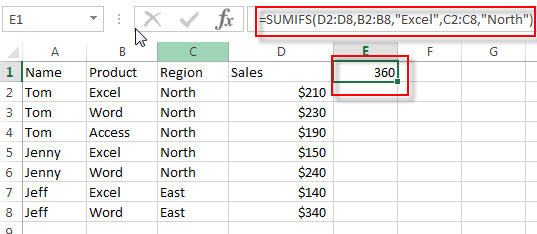# Excel SUMIFS Function

This post will guide you how to use Excel SUMIFS function with syntax and examples in Microsoft excel.

### Description

The Excel SUMIFS function sum the numbers in the range of cells that meet a single or multiple criteria that you specify. You can use the SUMIFS function to sum cells that based on the dates, numbers or texts based on one criteria in Excel. And it also supports logical operators and wildcards.

The SUMIFS function will extend the capabilities of the SUM function by giving you a choice that you want only sum those values that meet a single or multiple criteria.

The SUMIFS function is a build-in function in Microsoft Excel and it is categorized as a Math and Trigonometry Function.

The SUMIFS function is available in Excel 2016, Excel 2013, Excel 2010, Excel 2007, Excel 2011 for Mac.

### Syntax

The syntax of the SUMIFS function is as below:

`=SUMIFS (sum_range, criteria_range1, criteria1, [criteria_range2, criteria2], ...)`

Where the SUMIFS function arguments are:

• sum_range -This is a required argument. The actual cells to sum together.
• criteria_range1 -This is a required argument. The range of cells that you want to apply criteria1 against.
• criteria1 – This is a required argument. The criteria to use on criteria_range1.so this criteria defines which cells in criteria_range1 will be applied.
• [criteria_range2, criteria2] -This is an optional argument. It will be specified additional ranges and their associated criteria. Criteria2 will be applied against criteria_range2 and so on. It can be up to 127 criteria.

Note:

• Any criteria that include logical or math symbols must be enclosed in double quotation marks.
• The numeric criteria can be supplied without quotation marks.
• You can use the wildcard characters in the criteria argument.
• You can use multiple operators in the criteria argument.

### Excel SUMIFS Function Examples

The below examples will show you how to use Excel SUMIFS Function to sum the values in a range of cells based on multiple criteria.

Suppose that you have a sales table as shown below:

 Name Product Region Sales Tom Excel North \$210 Tom Word North \$230 Tom Access North \$190 Jenny Excel North \$150 Jenny Word North \$240 Jeff Excel East \$140 Jeff Word East \$340

1# to sum of the sales of the product “Excel” in region “North“, enter the following formula in Cell E1.

`=SUMIFS(D2:D8,B2:B8,"Excel",C2:C8,"North")`### Related Functions

• Excel SUM Function
The Excel SUM function will adds all numbers in a range of cells and returns the sum of these values. You can add individual values, cell references or ranges in excel.The syntax of the SUM function is as below:= SUM(number1,[number2],…)…
• Excel SUMIF Function
The Excel SUMIF function sum the numbers in the range of cells that meet a single criteria that you specify. The syntax of the SUMIF function is as below:=SUMIF (range, criteria, [sum_range])…
• Excel SUMSQ Function
The Excel SUMSQ function returns the sum of squares of a given set of arguments.The syntax of the SUMSQ function is as below:=SUMSQ(number1, [number2], …)…
• Excel SUMX2MY2 Function
The Excel SUMX2MY2 function returns the sum of the difference of squares between two supplied arrays.The syntax of the SUMX2MY2 function is as below:=SUMX2MY2 (array_x, array_y)…